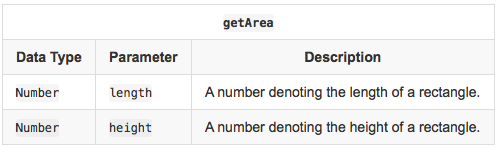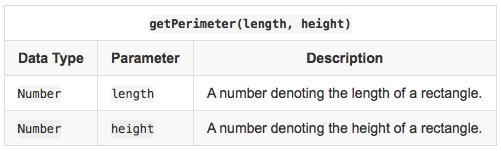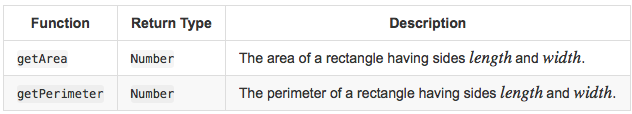# [Solved] HackerRank Javascript in 10 days - JS 10 days arithmetic operators solution in Javascript HackerRank

## Algorithm

We know the basic formula to get area and peremeter of a rectangle.

Formulas:

Area of a rectangle = length * width

Peremeter of a rectangle = 2 * ( length + width )

## Code Examples

### #1 Code Example with Javascript Programming

Code - Javascript Programming

/**
*   Calculate the area of a rectangle.
*
*   length: The length of the rectangle.
*   width: The width of the rectangle.
*
*	Return a number denoting the rectangle's area.
**/
function getArea(length, width) {
let area;

area = length * width;

return area;
}

/**
*   Calculate the perimeter of a rectangle.
*
*	length: The length of the rectangle.
*   width: The width of the rectangle.
*
*	Return a number denoting the perimeter of a rectangle.
**/
function getPerimeter(length, width) {
let perimeter;

perimeter = 2 * ( length + width );

return perimeter;
}

Copy The Code &

Input

cmd
3
4.5

Output

cmd
13.5 15

## Demonstration

Hello, this is a very simple calculation problem.

We know the basic formulas and it has also written there.

Details of the problem:

Objective

In this challenge, we practice using arithmetic operators. Check out the attached tutorial for resources.

Complete the following functions in the editor below:

1. getArea(length, width): Calculate and return the area of a rectangle having sides  and .
2. getPerimeter(length, width): Calculate and return the perimeter of a rectangle having sides  and .

The values returned by these functions are printed to stdout by locked stub code in the editor.

Input FormatConstraints

•  and  are scaled to at most three decimal places.

Output FormatSample Input 0

3
4.5


Sample Output 0

13.5
15


Explanation 0

The area of the rectangle is .

The perimeter of the rectangle is .